#### You may also likeFour rods, two of length a and two of length b, are linked to form a kite. The linkage is moveable so that the angles change. What is the maximum area of the kite?### Making Rectangles, Making Squares

How many differently shaped rectangles can you build using these equilateral and isosceles triangles? Can you make a square?This gives a short summary of the properties and theorems of cyclic quadrilaterals and links to some practical examples to be found elsewhere on the site.

##### Age 11 to 14Challenge Level

Many thanks to all of you who submitted solutions to this problem: you've used several different methods. Many of you used flow charts to present your solutions, which made it very easy to see what questions you were asking depending on the answers to your previous questions.

Mariah, Georgia and April, from Hastings Secondary College, Australia, submitted this solution. They used the fact that their kite and arrowhead had right angles in them to arrange their questions.

These are their shapes, and their questions that they would ask: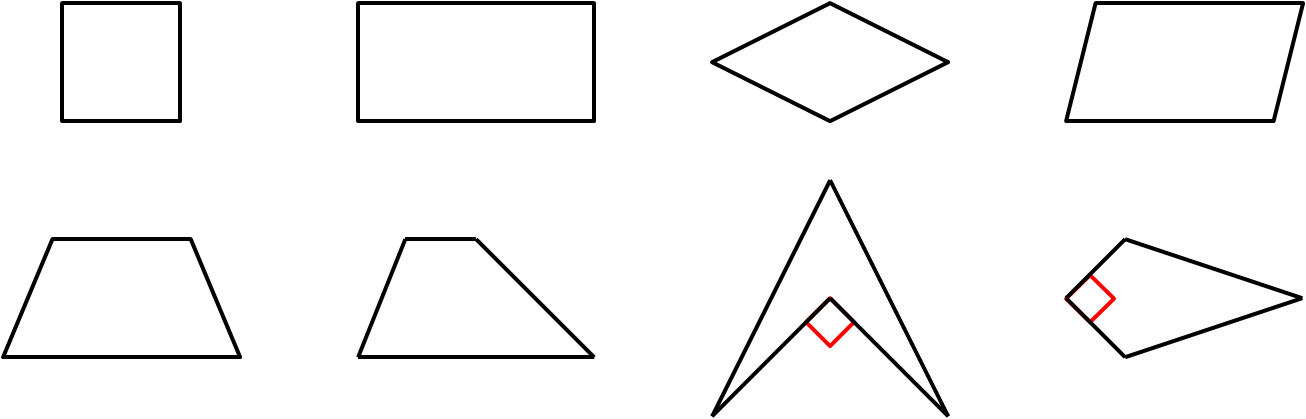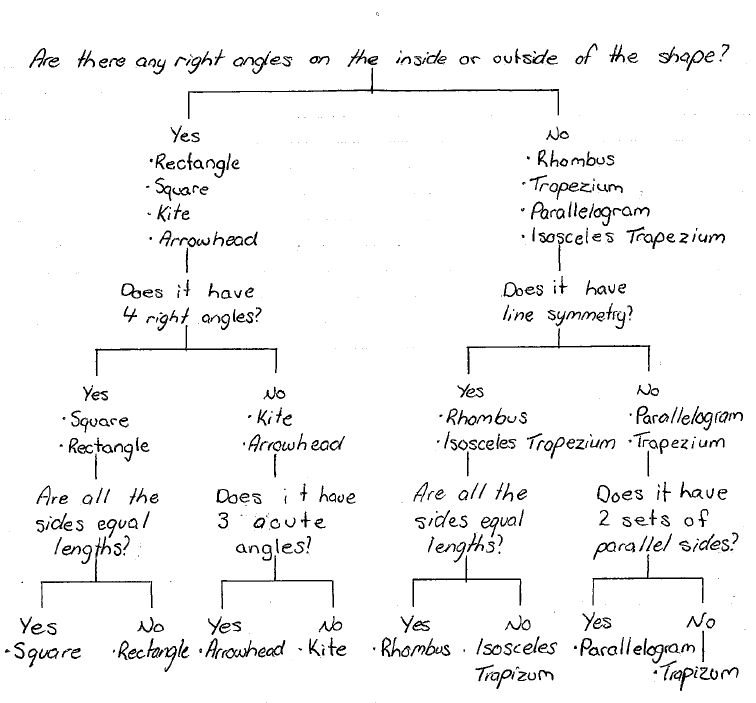Nathan, from Bishop Wordsworth's School, explained why three questions was the minimum that you would need to be able to work out what the quadrilateral is:

I realised that to get the smallest result, each question asked would have to halve the number of shapes left until you have the final shape. Therefore, the minimum amount of questions you could ask and certainly get a right answer is three: $8 \div 2 = 4$; $4 \div 2 = 2$ and $2 \div 2 = 1$, which makes a total of 3 questions.

He was also able to find a way to work out the shape using only three questions. He was able to do this without assuming any extra properties of the shapes: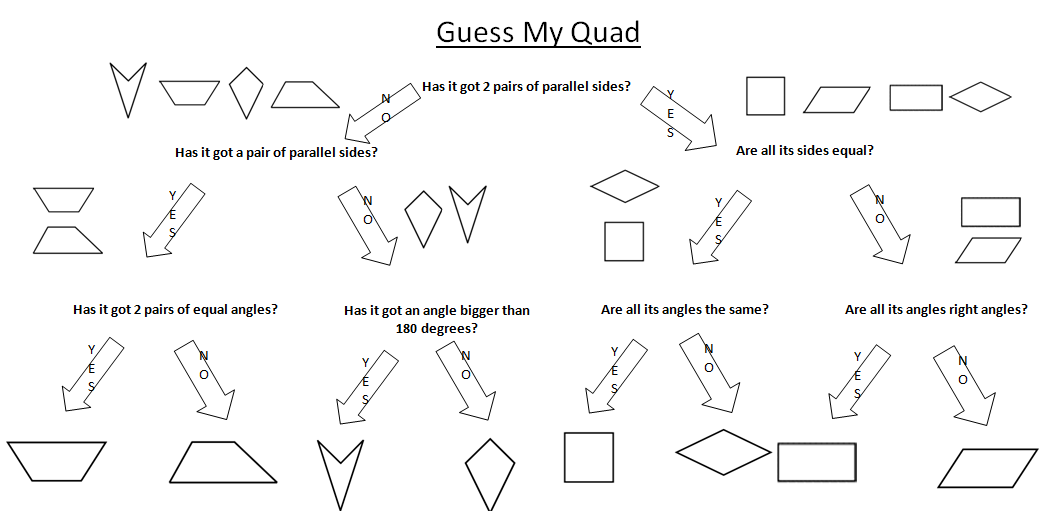Zach also had a flow chart to show his method: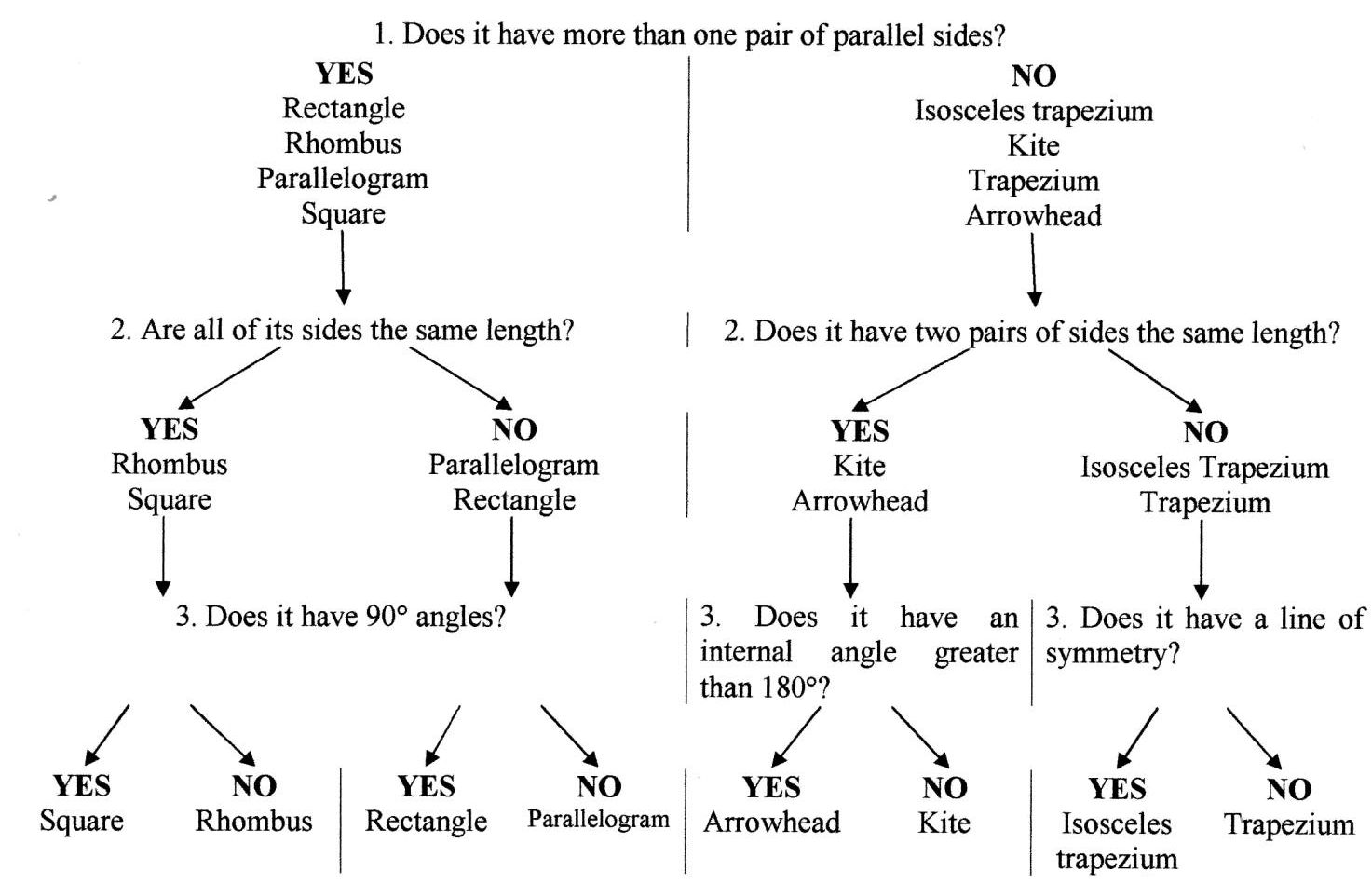He also extended this by adding some more shapes, which meant that he required four questions. He said that he needed eight new shapes, since each question halves the number of shapes. These are the shapes he added: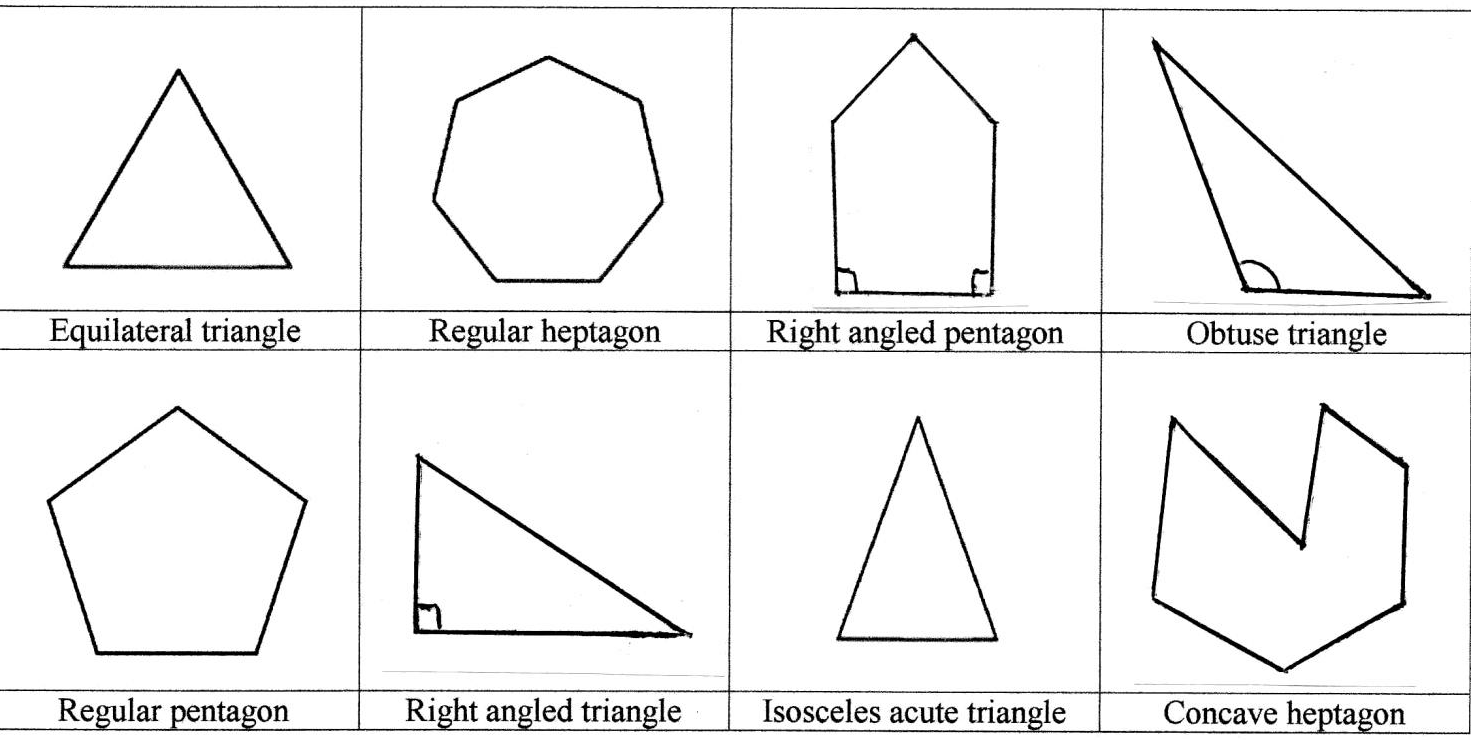He then suggested this set of questions: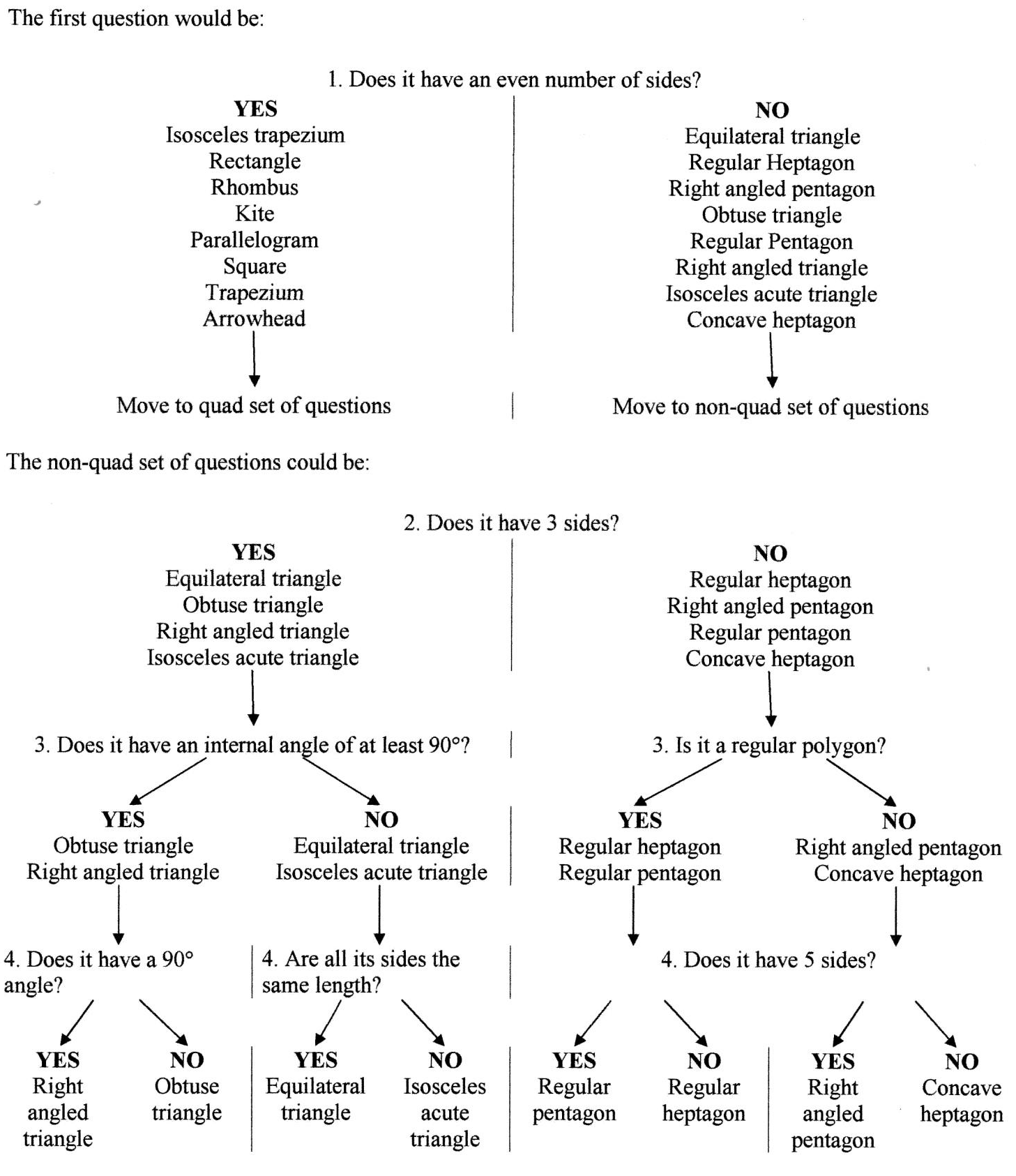Thank you and well done to everyone who submitted a solution.